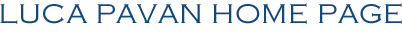MAIN DOS PROGRAMS:    MCCs 2    Cs_utilities :_________    Cs Pocket Manual    CSST :_________   SMU MUSIC:    Planet    Amber :_________ BioSMU - Sound Math Utils Sound utilities Windows command prompt         SMU is a group of small programs for Windows command prompt. Each program does audio calculations. The programs are standalone or can be used trough a menu. They should work on Windows 32 and 64-bit systems. Here is the list of programs: 1 AMP2DB It converts raw amplitude to dBs 2 DB2AMP It converts dBs to raw amplitude 3 AMP2DBFS It converts raw amplitude to dB in full scale (dBFS) 4 DBFS2AMP It converts dB in full scale (dBFS) to raw amplitude 5 WATT2DB It converts Watts to dBs 6 DB2WATT It converts dBs to Watts 7 DBISL It finds the amplitude [dB] according to the inverse square law 8 HZ2MIDI It converts Hz to MIDI numbers 9 MIDI2HZ It converts MIDI numbers to Hz 10 OVERT It calculates the frequency of overtones in periodic sounds 11 AM It calculates the sidebands frequency in AM synthesis 12 FM It calculates the sidebands frequency in FM synthesis 13 OCTAVE It divides the octave in equal parts 14 BEATS It calculates the beat frequency between two sine waves 15 CENTERBP It calculates the center frequency of a bandpass filter 16 ECHO It calculates the time interval between echoes 17 DOPPLER It calculates the frequency of Doppler shift 18 STWAVES It calculates the frequencies of standing waves in a room  size 604554 bytes   © 2001-2021 Luca Pavan